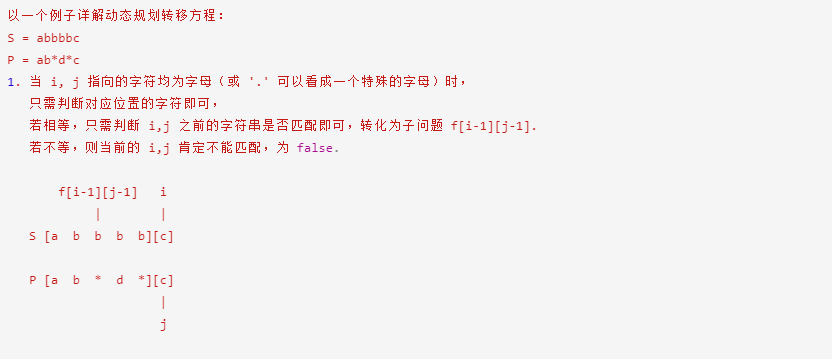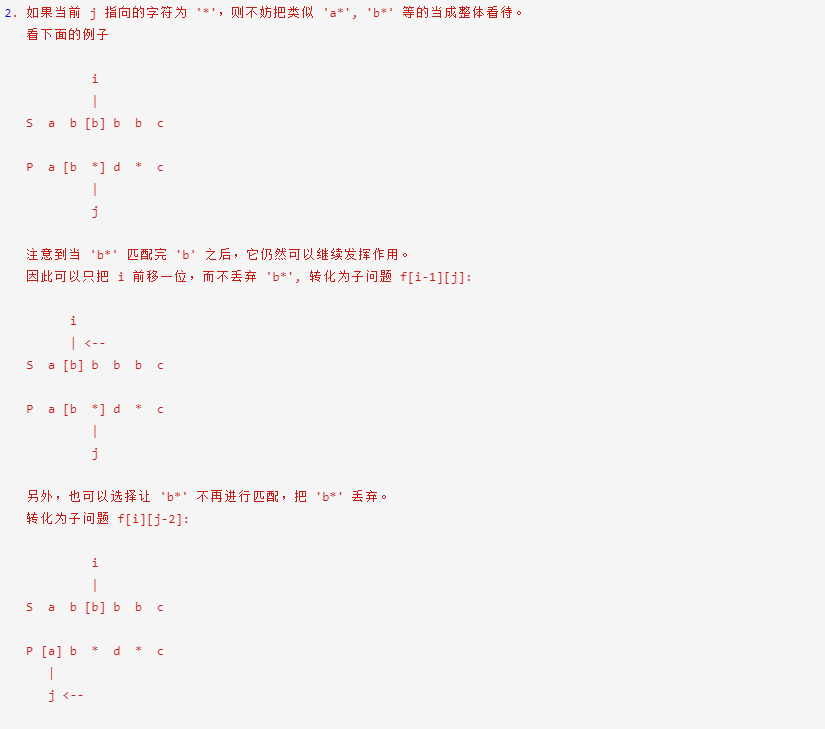## 【LeetCode 10】正则表达式匹配

### 错误版本

可以通过2/3的测试样例，发现每次判断当前字符的时候还需要判断后面一个甚至两个字符的情况。且代码有重复的情况，没有把各种情况完全归并到一起。没能很好的抽象问题。

``````public static boolean isMatch(String s, String p) {

boolean ret = false;
int m = s.length(); // for patter s
int i = 0;
int n = p.length(); // for patter p
int j = 0;
while (j < n)
{

char c = p.charAt(j);
if (c == '.' ) // 任意单个字符都可以通过
{

i++;
//j++;
}

else if (c == '*') // 任意多个字符
{

if (j-1 >= 0) // * 不可能出现在字符串的第一个
{

char bc = p.charAt(j-1); // 记录前一个字符
// 可以匹配多个任意字符，所以究竟匹配多少个呢？
// 假如匹配到结尾位置的话，
if (bc == '.') // 可以匹配多个任意字符，所以究竟匹配多少个呢？
{

// 方式1，整个字符串全部匹配完 no
// 方式2，只匹配几个字符，剩下的留给相同字符的进行匹配
if (j+1 < n) // 后面还有字符
{

char ac = p.charAt(j+1);
if (ac != '.') // 后面的字符是普通字符
{

while (i < m)
{

if (s.charAt(i) == ac)
break;
i++;
}
if (i == m) // 因为没有找到匹配的字符
return false;
i++; // 有相同字符，因此进入到下一个字符
}
else // 对于.来说，只要字符串后面还有字符，则是正确的。必须要匹配一个字符，这个字符就是j+1
{

j++;
i++;
continue;
}
}
else // 后面没有字符了, *可以匹配0个或多个字符
{

return true;
}
}
else // 同一个字符，直到出现不匹配停止匹配
{

/*
*  同样需要考虑有没有后续字符
*/
/*if (j+1 < n)
{
if (p.charAt(j+1) == s.charAt(i))
{
j++;
i++;
}
else if (j+2 < n && p.charAt(j+2) == '*')
{
j += 2;
}
}
*/
while (i < m) // 从这里退出，i表示没有相同字符
{

if (s.charAt(i) == bc)
break;// 从这里退出，则i表示相同字符
i++;
}
if (i == m) // 因为没有找到匹配的字符
return false;
i++;
}
}
}
else // 普通字符的情况
{

boolean flag = false; // false 表示是匹配字符
if (i < m && s.charAt(i) != c)
{

if (j+1 == n)
return false;
else if (p.charAt(++j) != '*')
return false;
flag = true;
}
if (!flag)
i++; // 情况1：有相同字符，因此进入到下一个字符
// 情况2：两个字符不相同，但是因为有* 不进入下一个字符，保留当前位置
// 情况3：后面没有字符，当前字符只能是失配字符 已经退出
}
j++;
}
if (i == m && j == n)
ret = true;
else
ret = false;
return ret;
}
``````

## 正确版本之动态规划``````public static boolean isMatch(String s, String p) {

int r = s.length(), c = p.length();
if (r == 0 || c == 0)
return false;
boolean[][] match = new boolean[r+1][c+1];
// 初始化第一列
match = true;
for (int i = 1; i < r+1; i++)
match[i] = false;
// 初始化第一行
for (int j = 1; j < c+1; j++)
{

match[j] = false;
if (j-2 >= 0)
{

int p_p = j-1; // 访问的是第j个字符
if (p.charAt(p_p) == '*' && match[j-2] == true)
match[j] = true;
}
}
// p和s匹配或者，p的当前字符是.
for (int i = 1; i < r+1; i++)
for (int j = 1; j < c+1; j++) // 先按照模式串（列）循环，再按照原来的字符串（行）循环
{

int p_p = j-1; // 字符串中的访问位置，实际代表的是第j个字符
int s_p = i-1;
if (p.charAt(p_p) == s.charAt(s_p) || p.charAt(p_p) == '.') // 当前字符相同或者模式串中有"."
match[i][j] = match[i-1][j-1]; // 匹配表中的情况
else if (p.charAt(p_p) == '*')  // 第j个字符是*
{

if (j-2 >= 0) // 数组访问的位置
{

if (p.charAt(p_p-1) == s.charAt(s_p) || p.charAt(p_p-1) == '.')  // 第j-1个字符和第i个字符的匹配情况，用p_p和s_p表示字符串的位置
{

match[i][j] = (match[i][j-2] || match[i-1][j]);
}
else
{

match[i][j] = match[i][j-2]; // 删除第j个字符后之前字符串的匹配情况
}
}
}
else
{

match[i][j] = false;                 // 当前字符不相同
}

}
return match[r][c];
}
``````

### Guess you like

Origin blog.csdn.net/juttajry/article/details/121424296
Recommended
Ranking
Daily